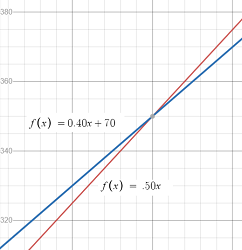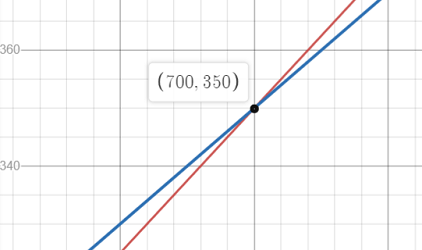# Break Even Point (Calculus)

The break even point is where revenue equals cost. In other words, it’s when these two functions are equal, so we can find the break even point at the intersection of the cost and revenue functions.

Algebraically, you could set R(x) = C(x) and solve for x. Or, you could set the profit function equal to zero and solve for x. In calculus though, we usually skip the algebra and head straight for a graph, which can be much easier for ugly-looking functions.

If there are two points or more, you’re generally only concerned with the first point: the point at which the break-even point is first achieved.

## 1. Break Even Point: Graph Example

Example question: A phone app has fixed production costs of \$70 for the cover design and marginal distribution costs of 40 cents per copy. If each app sells for 50 cents per download, what is the break even point?

Step 1: Find the Revenue function. The company is selling the app for \$0.50, so the revenue function is:
R(x) = 0.50x.

Step 2: Find the Cost function. The question states that the marginal cost is \$0.40 per copy, and the fixed costs are \$70. This
means that the cost function is:
C(x) = 0.40x + 70.

Step 3: Graph the two functions.Step 4: Find the Intersection. Exactly how you find the intersection (the crossing point) depends on your graphing calculator. I’m using Desmos here, and to find the intersection just click on the point where the two graphs cross and it will show you the coordinates.If you have a TI-89 see How to graph two functions and find the intersection on the TI-89..
The coordinates are (700, 350).

Step 5: Identify the “x” value in the coordinates from Step 4. This is the break even point: the “x” units that must be sold to break even.

Break even point = 700 units.

That’s it!

## Break Even Point: Algebra Example

Example question: A magazine publisher reports that their revenue function and cost functions (in thousands of dollars) are:

• R(x) = 32x – .21x2
• C(x) = 195 + 12x.

How many subscribers does the publisher need to break even?’

Step 1: Find the Profit Function:
Profit = Revenue – Cost, so:
Profit = (32x – .21x2) – (195 + 12x)

Step 2: Set the formula from Step 1 to zero:
0 = (32x – .21x2) – (195 + 12x)

Step 3: Solve for x. This particular solution requires the quadratic formula with a= 195, b = 20 and c = .21 (I used Symbolab’s “solve by quadratic formula” option here).We’re want the earliest break even point, which for this example is:
11.03 thousand magazine subscribers.